# New to Qlik Sense

Discussion board where members can get started with Qlik Sense.

Contributor

## How to address chart's dimension in the expression (set analysis)

Hi everyone,

so there's the issue I've been struggling with (so would appreciate any help)

I have such expression in a bar chart:

Which basically shows the sum of trans_value but only for buyers with no less than 10 transaction.

Then I need to implement this solutions to another two charts:

1) Has alternative dimensions (main is Date, alternative is Month and Year)

2) Has drill down (product category)

Is there any syntax to not address dimension in set analysis directly, but rather point to a current chart Dimension (something like when Color by Dimension is selected and "Dimension 1" can be used as the field)?

Somewhat like sum({<\$Dimension1={"=count(trans_id)>=10"}>}trans_value)

Or maybe there's a function which will return the current chart dimension?

5 RepliesPartner

## Re: How to address chart's dimension in the expression (set analysis)

Please try to refer to this:

https://community.qlik.com/message/636642?&_ga=2.225612829.641101863.1535966298-1574998573.153596629...

Especially Jonathan response:

###### Re: QLIK SENSE: \$(=GetCurrentField(Time))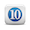Paulo ,  currently the getcurrentfield() isn't valid in Sense .

Would you be interested in a potential workaround ?

Its a little klugy instead of using getcurrentfield() i'm using a count(distinct total) to check how many field values are within my selection.

Say you have Country->City->Customer drill path and at the top level (when viewing countries) you want the pie to count the number of cities.  When a user drills on one country and narrows the selection to just one country to see the cities within that country, then they want the measure to count the customers in each city.

Here is a conditional expression (think of it as long form version of getcurrentfield() )  to figure out which level of the hierarchy you are in.

In this example, the lowest level (customer) also shows the number of customers which doesn't make much sense but ... its just a quick example, you could add a nested IF to count ( distinct total city) = 1 and then display sum(SAles) or another metric at the lowest level.

Would this work for you ?

if (count( distinct total Country) > 1, Count(distinct City) , if ( count( distinct Country)=1 , Count(distinct Customer)))

Here is a 3 step drill visual of this in action: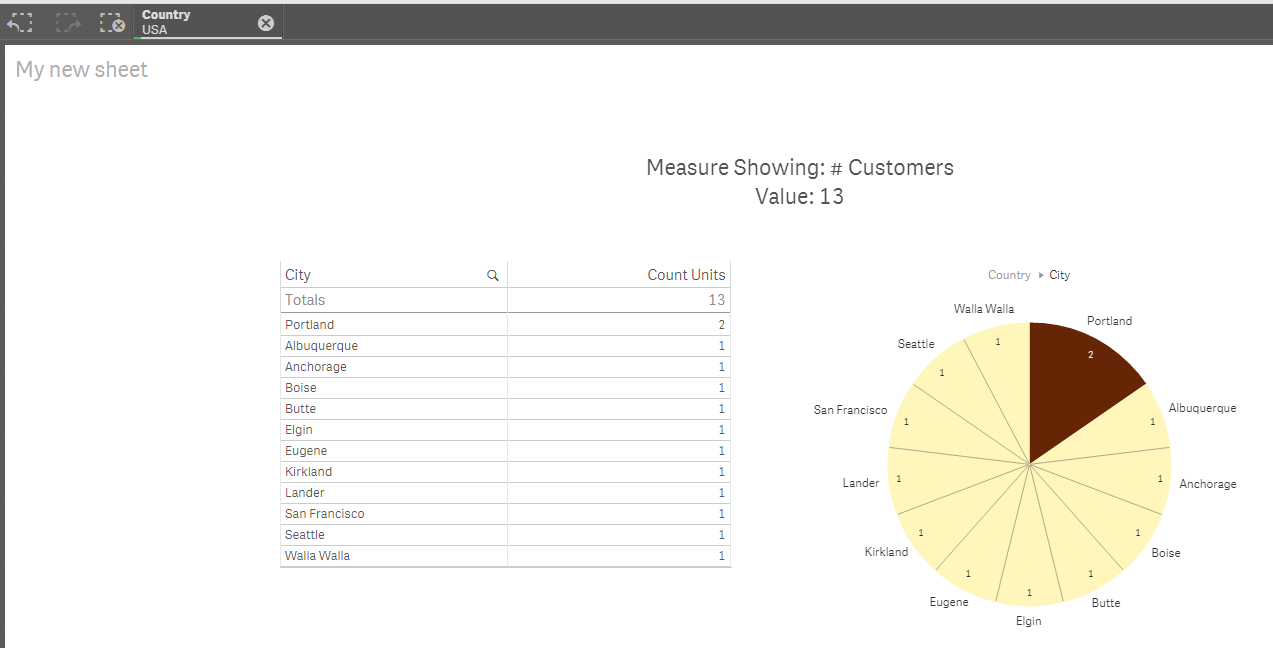Partner

## Re: How to address chart's dimension in the expression (set analysis)

You can also try to follow alvaro's logic:

###### Re: GetCurrentField() in Qlik sense  ?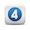Hi Satish,

It'd be great to have GetCurrentField function in Qlik Sense, unfortunately it's still not available.

Let me know what you think about this workaround using GetSelectedCount, which is supported in Qlik Sense. In my app I've created a drill-down group with the following time dimensions:

• Year
• Quarter
• MonthName
• Date

So, first of all, create a variable with the fields included in your drill-down group as follows:

vGetCurrentTimeFields = if(getselectedcount(Year)=0,'Year',

if(getselectedcount(Year)=1 and getselectedcount(Quarter)=0,'Quarter',

if(getselectedcount(Quarter)=1 and getselectedcount(MonthName)=0,'MonthYear',

if(getselectedcount(MonthName)=1 and getselectedcount(Date)=0,'Day',

if(getselectedcount(Date)=1, 'Day')))))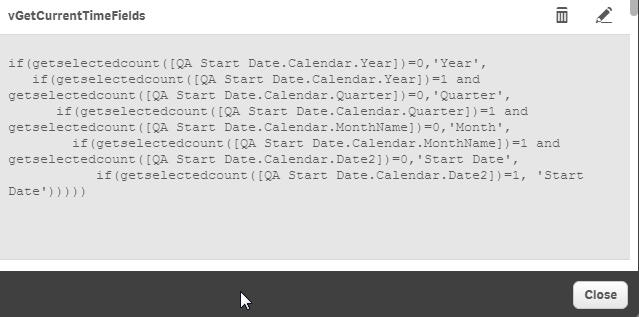I know what you might be thinking... field names are hardcoded. Yes, but with this simple logic you can retrieve the current field name within a drill down group!! Look at the following pictures. I created a bar chart in which the title is dynamic: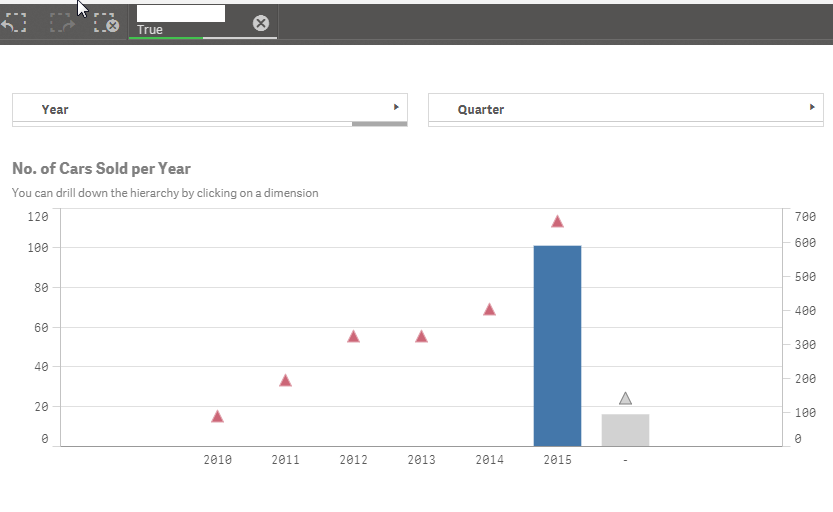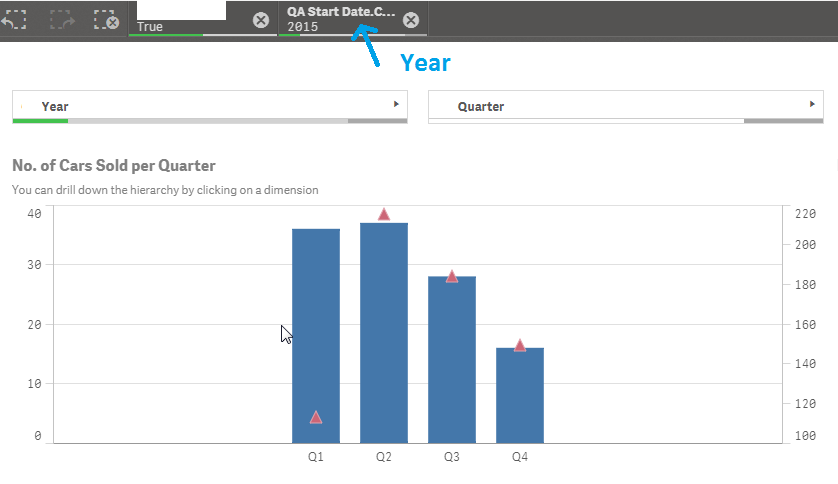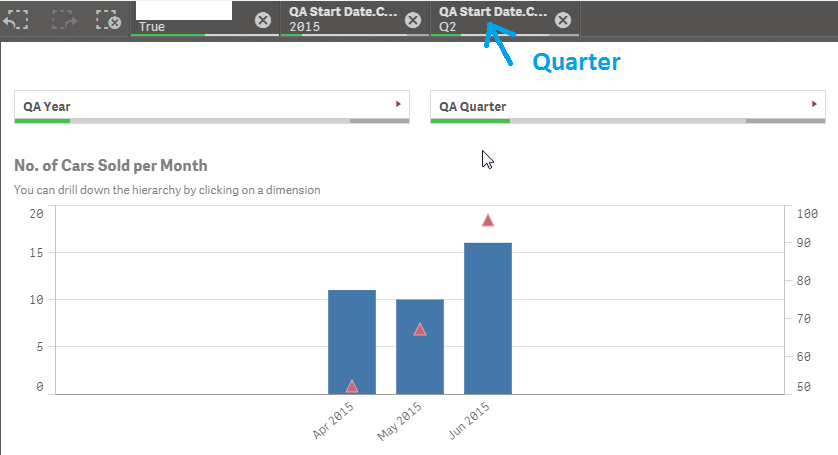Hope this was helpful!

Alvaro P.

Highlighted
Contributor

## Re: How to address chart's dimension in the expression (set analysis)

Thank you Omar!

I'll try both workarounds and see how each performs in set analysisPartner

## Re: How to address chart's dimension in the expression (set analysis)

Yet another solution, use of inline island table instead of alternative dimensions:

see this example and adjust it to ur need:

###### Re: Get active dimension from alternate dimensionWhat I would do is :

Creating an inline table:

For example:

Load * inline [

Dimensions

Article

Customer

]

;

Now, In my presentation,

I'll have a selection field: Dimensions

and in my chrt, as a dimension:

If(Dimensions='Article', "My Article Dimension",

if(Dimensions='Customer', "My Customer Dimension"))

and as a title:

='Dimension is : '&Dimensions:

Result: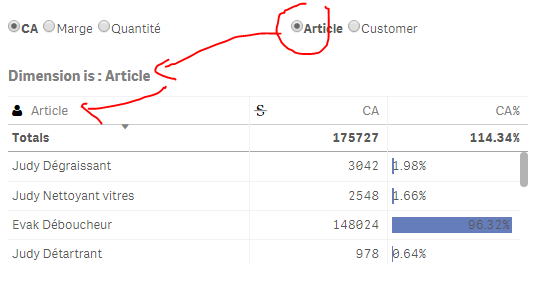Contributor

## Re: How to address chart's dimension in the expression (set analysis)

Hi Omar, here's an update on the matter:

There is actually a function, which allows you to reference Object's active field:  https://help.qlik.com/en-US/sense/June2018/Subsystems/Hub/Content/Scripting/SystemFunctions/GetObjec...

In my case it works in both cases (alternative and drill-down) with pick(match( :

=pick(match(GetFieldObject(0), '[FieldName1]', '[FieldName2]'),

expression1,

expression2

)